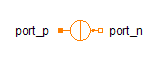Ramp - MapleSim Help

Ramp

Generate ramp signalDescription The Ramp component generates a continuous, real, ramp signal. The boolean parameter Smooth corners selects whether the corners are smoothed, the smoothness parameter, which can be varied from 0 to 1, determine the smoothness. If smoothness is greater than 0, the output signal has a continuous derivative. The smoothing interval is equal to the smoothness factor times half the ramp time.Equations If Smooth corners is false, the output signal is $y=\mathrm{offset}+\mathrm{height}\left\{\begin{array}{cc}0& t\le {T}_{0}\\ \frac{t-{T}_{0}}{{T}_{r}}& t\le {T}_{0}+{T}_{r}\\ 1& \mathrm{otherwise}\end{array}$ If Smooth corners is true, it is $y=\mathrm{offset}+\mathrm{height}\left\{\begin{array}{cc}0& t\le {T}_{0}\\ {\left(\frac{t-{T}_{0}}{{T}_{r}}\right)}^{2}\left(\frac{2}{S\left(2-S\right)}\right)& t\le {T}_{0}+S\frac{{T}_{r}}{2}\\ \frac{1}{2}+\left(\frac{t-{T}_{0}-\frac{{T}_{r}}{2}}{{T}_{r}}\right)\left(\frac{2}{2-S}\right)& t\le {T}_{0}+{T}_{r}-S\frac{{T}_{r}}{2}\\ 1-{\left(\frac{t-{T}_{0}-{T}_{r}}{{T}_{r}}\right)}^{2}\left(\frac{2}{S\left(2-S\right)}\right)& t\le {T}_{0}+{T}_{r}\\ 1& \mathrm{otherwise}\end{array}$ with $S=\mathrm{min}\left(1,\mathrm{max}\left(0,\mathrm{smoothness}\right)\right)\phantom{\rule[-0.0ex]{1.0ex}{0.0ex}}\mathrm{and}\phantom{\rule[-0.0ex]{1.0ex}{0.0ex}}t=\mathrm{time}$Connections

 Name Description Modelica ID $y$ Real output signal yParameters

 Name Default Units Description Modelica ID $\mathrm{height}$ $1$ Height of ramp height ${T}_{r}$ $s$ Durations of ramp duration Smooth corners $\mathrm{false}$ True means enable the smoothness factor smoothCorners $\mathrm{smoothness}$ Smoothness factor (0: sharpest, 1: smoothest) smoothness $\mathrm{offset}$ $0$ Offset of output signal offset ${T}_{0}$ $0$ $s$ Output = offset for time < startTime startTime